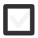# Can appreciate weight in everyday situations. Milk. The car. Person. 2 Ton-> Kg-> g+1 vote
914 views

## 2 lessons

+1 vote

Estimating the weight of different objects can be successfully done if we compare them to an object of the same type, or a similar type made of the same materials.

Milk is an interesting example.
Milk is a liquid and like most everyday liquids it consists almost entirely of water - 87%, the rest is protein, milk fat, lactose, micronutrients like calcium etc. So we will estimate that milk is a little bit heavier than water. One liter of water is exactly one kilogram in weight, that is the density of water -  1 kg/l. So we can say that a liter of milk has slightly the same density (weight per kilogram), maybe a little above but close.If we check it out it turns out one liter of milk is 1 kilogram and 30 grams, so for smaller amounts we can even approximate the weight of milk with a weight of water.
In fact we can approximate the weight of any liquid this way as long as it has similar density as water, we can estimate the density by examining the thickness of the substance though this is not entirely correct but for an estimation it is ok.

So a two liter bottle of juice, for example will most likely weigh around 2 kilograms as juices are almost entirely water.

CONVERSION - METRIC UNITS - t -> kg -> g

Like with all metric units, conversion factor between units of mass has a base of 10, or better say it is actually 103 = 1000. This means that like with units of length where 1 km = 1000 m, one 1 kg = 1000g. Prefix KILO in kilo-meter and kilo-gram, actually stands for 1000 in ancient greek.
So in the case of mass 1 tonn = 1000 kilograms and 1 kilogram = 1000 grams. If you are wondering are there any smaller units between tonn and kilogram, the answer is yes, we have unit such as decagram = 10 grams. Prefix DECA is derrived from latin and similar ancient greek work deca which means ten. We just don't see this unit very often since these days we find it unpractical, as most of the time we either measure things efficiently enough in kilos or grams.Are there smaller units than gram? Of course just like we have centimetar and milimetar we have centigram and miligram. Just like 1 metar = 100 cm, so will 1 g = 100 cg (centigrams) and 1 g = 1000 mg. But these units again we rarely use in everyday life. Mligrams you will most commonly find on medicine packages since medicines need to be administered in very small doses.

So how can we convert mass units than? It is easy just like with every other unit conversion when we convert from larger unit to smaller one we multply and when we convert from a smaller one to a larger one we devide. For each step of conversion we multiply or devide once.
In case we convert 2 tonns to grams we will proceed like this:

2 t X 1000 = 2000 kg X 1000 = 2 000 000 g

So between tonns and grams we had two steps of conversion and for every step we multiplied by 1000, if we would go the opposite way and convert from grams to tonns we would devied two times by 1000.

<!--[if gte mso 9]> <![endif]--><!--[if gte mso 9]> Normal 0 false false false EN-US X-NONE X-NONE <![endif]--><!--[if gte mso 9]> <![endif]--><!--[if gte mso 10]>

<![endif]-->